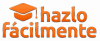# How the probability of something happening is calculated

Learn the basic formula of the calculation of probabilities, a very simple rule that can help you make your decisions better.How do you calculate the probability of something happening? Faced with different possible outcomes of an event, what if they don't have the same chances of happening?

The calculus of probabilities is one of the branches of mathematics that has been studied the most for centuries. Surely that is because, far from being something theoretical and abstract, it is a knowledge that helps us in our day to day.

This theory can help us make important decisions, for example when weighing the risks of making an economic investment. But we can also perform probability calculations just for fun and curiosity.

The probability that something happens is calculated in a very simple way according to the so-called Laplace rule: the probability that something happens (A) is equal to the number of favorable results divided by the possible results.

A = no. Favorable results / no. Possible results

We will multiply the result by 100 to know what percentage we are talking about.

The first thing we must do is distinguish between favorable results and possible results.

Given an event, for example, the throwing of a die, the possible results would be 6, since we can roll 1, 2, 3, 4, 5 or 6. The favorable results will be those that we choose, for example, the probability of rolling a 2; in this case we only have a favorable result, since if we roll a die we can only roll a 2 once.

If we apply the formula described above, we see that the probability of rolling a 2 when rolling a die is 16'67%.

Probability of taking 2 = 1 favorable result / 6 possible results = 1/6 = 0'1667

0'1667 x 100 = 16'67%

Favorable results don't always have to be 1, they can be more. For example, let's imagine that I have a bag with 10 white balls and 2 red balls, and I want to know what probability I have of drawing a red ball. In this case, we have 2 chances to be successful (get the red ball), therefore, there are 2 favorable outcomes out of 10 possible (total balls).

A = 2/10 = 0.2 x 100 = 20% of probabilities of drawing a red ball

Another example. In the toss of a coin, the probability of getting heads or tails is 50% (1/2 = 0.5 x 100 = 50%), but I'm going to think that I have two coins. I want to know what probability I have of getting heads and tails.

By tossing the 2 coins I can get: heads and tails, tails and heads, heads and heads, or tails and tails. Here I have 4 possible results and 2 favorable ones (that the coins do not get the same result). Therefore:

2/4 = 0.5 x 100 = 50%

Of course, things can get more complicated. In the case of a coin, I only have 2 possible outcomes, but with a die I have 6. So if we want to calculate the probabilities that a certain number will come up by adding the result of the roll of two dice, we have to do many more calculations. . For example, we have a 2'78% (1/36) to get a 2 or a 12, and a 8'33% (3/36) to obtain a sum of 4 or a 10.

In all this calculation of probabilities applying Laplace's rule there is something very important that we must always keep in mind: all possible results have the same chances of being produced.

This, of course, is not always the case. In real life, there are events that are more likely to occur than others. In a soccer match, for example, there are always 3 possible outcomes: home win (1), draw (X) or away win (2). However, we all know that 1, X and 2 do not have the same chances of occurring. If Real Madrid face Recreativo de Huelva at home, it is very likely that Madrid will win.

Here many other factors come into play when calculating the probability, something that brokers, meteorologists or brokers usually work with. responsible for setting forecasts sports They use parameters that are based on observation or statistics to perform their calculations. Various information is taken into account to establish the probability of a result occurring.

Odds for football matches or other events are nothing more than the result of those betting house odds calculations. However, they are shown in an inversely proportional way: the lower the odds, the more likely an event will happen (therefore, if we are right, we will win less). For example, at an odds of 1.10 for Real Madrid to win, there is a probability of 90% (approximately) that this result will occur. Instead, a quota of 100 would have a probability of 1%.

In mathematics, one of the methods of doing these calculations is by observing the results after several repetitions. There is talk of relative frequency, that is, when an event occurs a certain number of times, see in how many of them we obtain a favorable result. For example, let's imagine that Real Madrid and Recre have met 20 times throughout their history, and Madrid have won 15 of those times; this result will be a factor that helps us determine that Madrid's probability of victory is much higher than that of Recre. The more factors we study, the better we can establish the probability.

## Other ways to do it easily

#### Article information

This website uses Facebook pixel data and cookies to track our marketing and traffic efforts so that we can better serve you. Learn more